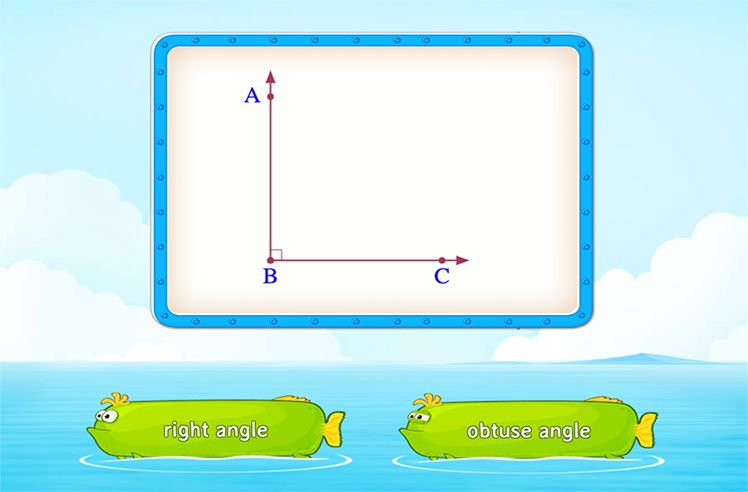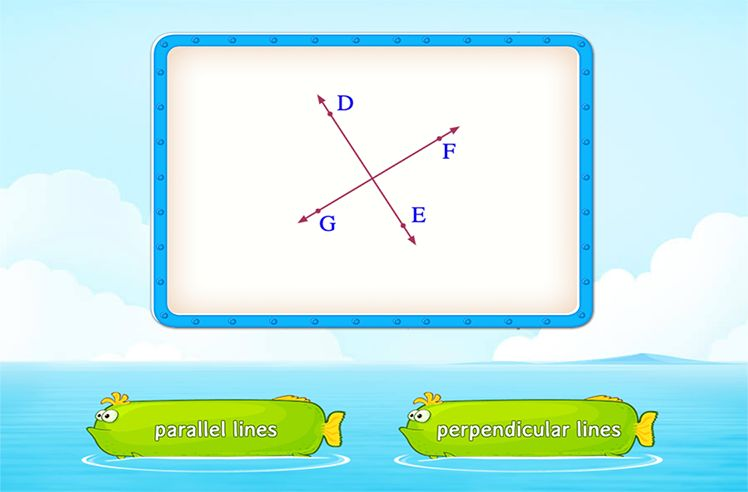# 30 60 90 Triangle – Definition with Examples

» 30 60 90 Triangle – Definition with Examples

A three sided polygon is known as a triangle. A triangle has 3 sides, 3 vertices and 3 angles. There are different ways in which we can categorize the triangles. On the basis of sides, a triangle can be scalene, isosceles, or equilateral. However, in some cases, a triangle may possess unique properties. Such triangles are called special triangles. One such special triangle is the 30°–60°–90° triangles.

## What Is a “30-60-90” Triangle?

A special right triangle with angles 30°, 60°, and 90° is called a 30-60-90 triangle. The angles of a 30-60-90 triangle are in the ratio 1 : 2 : 3. Since 30° is the smallest angle in the triangle, the side opposite to the 30° angle is always the smallest (shortest leg). The side opposite to the 60° angle is the longer leg, and finally, the side opposite to the 90° angle is the largest side of the right triangle, also known as the hypotenuse.

Since the 30-60-90 triangle is a special triangle, the side lengths of the 30-60-90 triangle are in a constant relationship.

From the figure above, we can make the following observations about the side length ratio of a 30-60-90 triangle:

• The side opposite the 30° angle: PQ = a
• The side opposite the 60° angle: QR = a√3
• The side opposite the 90° angle: PR = 2a

The sides of a 30-60-90 triangle are always in the ratio of 1 : √3 : 2.

For example:

Here, in triangle PQR,

• The side opposite to the 30° angle is PQ = a = 5 units
• The side opposite to the 60° angle is QR = a√3 = 5√3 units
• The side opposite to the 90° angle is PQ = 2a = 10 units

## 30-60-90 Triangle Rule

In a 30-60-90 triangle, we can find the measure of any of the three sides by knowing the measure of at least one side in the triangle. This is known as the 30-60-90 triangle rule. The following shows how to find the sides of a 30-60-90 triangle using this rule:

• When the side opposite to 30° is given.

Consider the side opposite to 30° (shortest side), AB to be ‘a’.

The side opposite to 60°, BC = a√3

The side opposite to 90° (hypotenuse), AC = 2a

• When the side opposite to 60° is given.

Consider the side opposite to 60°, EF to be ‘a’.

The side opposite to 30°, DE = $\frac{a}{\sqrt{3}}$

The side opposite to 90° (hypotenuse), DF = $\frac{2a}{\sqrt{3}}$

• When hypotenuse is given.

Consider the hypotenuse of the triangle, i.e., PR to be ‘a’.

The side opposite to 30°, PQ = $\frac{a}{2}$

The side opposite to 60°, QR = $\frac{\sqrt{3}}{2}$a

## Area of the 30-60-90 Triangle

When the perpendicular is given to be a,

Area of $\Delta$DEF = $\frac{1}{2} \times base \times height$

= $\frac{1}{2} \times a \sqrt{3} \times a = \frac{\sqrt{3}a^{2}}{2}$

## Conclusion

In this article, we learned about a special triangle, i.e., the 30-60-90 triangle. To read more such informative articles on other concepts, visit our website. We, at SplashLearn, are on a mission to make learning fun and interactive for all students.

## Solved Examples

1. Find the length of the side BC in the following figure.

Answer: The side opposite to 30˚ (shortest side), AB = 6 cm

Since the sides of a 30-60-90 triangle are always in the ratio of 1 : √3 : 2.

Therefore, the sides of the given triangle are AB = 6 cm, BC = 6√3 cm and AC = 12 cm.

So, BC = 6√3 cm

2. Find the length of the hypotenuse in the following figure.

Answer: Side opposite to 60˚, QR = a = 8√3 cm

The hypotenuse of the triangle, i.e., PR = $\frac{2a}{\sqrt{3}} = \frac{2 × 8√3}{\sqrt{3}}$ = 16 units.

3.  A triangle has sides 3√2, 3√6, and 3√8. Find the angles of this triangle.

Answer: The sides of the triangle are 3√2, 3√6, and 3√8.

Let us check whether the sides are of the 30-60-90 triangle.

On dividing each side of the triangle by 3√2, we get 1, √3, and 2. Therefore, sides of the triangle are 3√2, 3√6, and 3√8, are in the ratio 1: √3: 2.

The sides are following the 30-60-90 triangle rule. So, the angles of the given triangle are 30°, 60°, and 90°.

## Practice Problems

### 1Which of the following forms a 30-60-90 triangle?

5, 5√3, 10
5, 5√3, 20
10, 5√3, 10
None of these
CorrectIncorrect
Correct answer is: 5, 5√3, 10
On dividing the ratio 5 : 5√3 : 10 by 5, we get 1 : √3 : 2. It follows the 30-60-90 triangle rule.

### 2The hypotenuse of a 30-60-90 triangle as shown below is 20 ft. Find the length of the shortest side of the triangle?

10 feet
10$\sqrt{3}$ feet
15
$\frac{10 \sqrt{3}}{3}$ feet
CorrectIncorrect
In a 30-60-90 triangle, the length of the hypotenuse is twice the length of the shortest side. So, the shortest side of the triangle must be $\frac{hypotenuse}{2}$ = $\frac{20}{2}$ = 10 ft

### 3What will be the area of the triangle if the base is given to be 4√3 cm?4 cm$^{2}$
4$\sqrt{3}$ cm$^{2}$
8 cm$^{2}$
8$\sqrt{3}$ cm$^{2}$
CorrectIncorrect
Correct answer is: 8$\sqrt{3}$ cm$^{2}$
Base is 4√3 cm and perpendicular must be 4 cm. So, Area of the triangle = $\frac{1}{2} \times 4 \times 4√3 = 8√3 cm^{2}$

These are some similarities between the 30-60-90 triangle and 45-45-90 triangle.

• Both are right-angle triangles.
• Sum of the interior angles of both are 180 degrees.

Let the perpendicular be a.

Base = 3a

Hypotenuse = 2a Perimeter = Sum of all the sides = a+3a+2a = a(3+3) units

The long leg of a 30-60-90 triangle is the one whose length is greater than the shortest leg and less than the hypotenuse. In other words, it is the side opposite the 60 degree angle.Shapes

Play NowAngles

Play NowParallel and Perpendicular Lines

Play Now
[class^="wpforms-"]
[class^="wpforms-"]
[class^="wpforms-"]
[class^="wpforms-"]